# How to use the ATAN Function in Excel

In this article, we will learn about how to use ATAN function in Excel.

TAN function is a trigonometric function to calculate the tangent of the angle of a right angled triangle. Tangent value of an angle of the triangle returns the ratio of the sides i.e. opposite site ( = y ) / adjacent site ( = x ).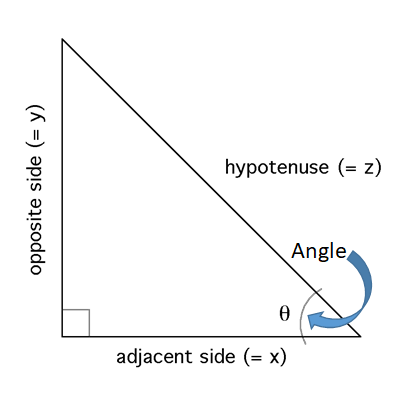tangent of an angle is the angle made by the side and hypotenuse
Tangent (Angle()) = opposite site ( = y ) / adjacent site ( = x )
TAN () = x / z
So, it means tangent function returns the ratio of the sides when the angle is given as input. Now if we wish to return the angle when ratio of the sides is given as input, we’ll use the inverse of the tangent function which is the ATAN function. The above explained representation is shown in below equations
=  TAN -1( x / z ) { in mathematics }
=  ATAN ( x / z ) { in Excel
ATAN function in excel returns the angle value in radians, the function takes input the ratio of the two sides.

Syntax:

 = ATAN ( number )

number : basically the ratio of the two sides in radians. number of the input argument can be any number.
Let’s understand more about the ATAN function using it in an example: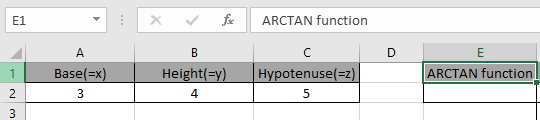Here we have the lengths of sides of the right - angle Triangle having sides as base, height and hypotenuse.
So we need to find the inverse tangent of the ratio of the sides.
Use the formula:

 =ATAN(A2/C2)

A2/C2 : it returns the ratio of the sides where value of the sides is given in as cell reference.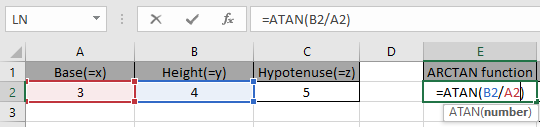Given the ratio of the sides as input to the ATAN function in excel and Press Enter.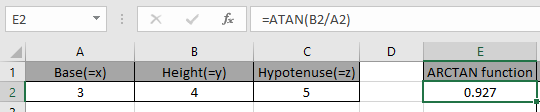As you can see, the ATAN function returns a value in radians.

The above method returns the value in radians. So to convert the value from radians to degrees use the DEGREES function or multiply the result with 180/PI().
Use the formula:

 = DEGREES ( ATAN ( E2 )

OR

 = TAN ( E2 ) * 180 / PI()The above snapshot gets the result as 53.13 degrees.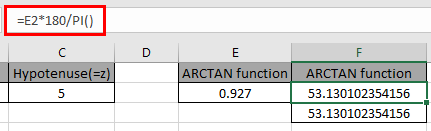The above snapshot use the DEGREES function which takes the input in radians and returns the value in degrees.

Here are some of the observations gathered using the ATAN function.

Note:

1. The function returns in the range of -1.57.. ( -90 degrees ) to +1.57.. ( 90 degrees ).
2. The function returns #VALUE! Error if the argument to the function is non numeric.

As you can see, we can get the inverse of the tangent function using ATAN function.

Hope you understood How to use ATAN function in Excel. Explore more articles on Mathematical function like ASIN function and ACOS function here. Please state your query in the comment box below.

Terms and Conditions of use

The applications/code on this site are distributed as is and without warranties or liability. In no event shall the owner of the copyrights, or the authors of the applications/code be liable for any loss of profit, any problems or any damage resulting from the use or evaluation of the applications/code.